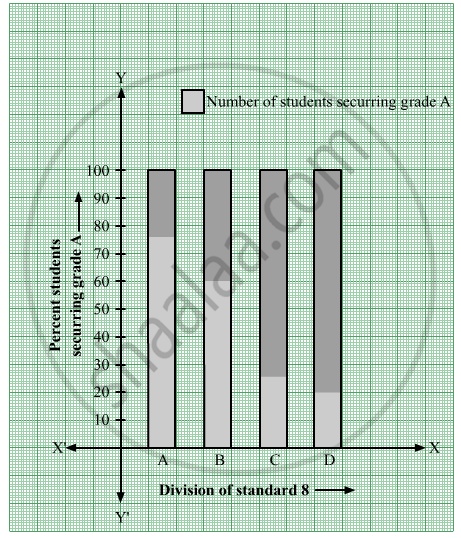# Show the Following Information by Percentage Bar Graph. - Mathematics

Graph

Show the following information by percentage bar graph.

 Division of standard 8 A B C D Number of students securring grade A 45 33 10 15 Total number of students 60 55 40 75

#### Solution

We have,

The following information:

 Division of standard 8 A B C D Number of students securring grade A 45 33 10 15 Total number of students 60 55 40 75 Percentage of students securring grade A 45/60 xx 100 = 75 33/55 xx 100 = 60 10/40 xx 100 = 25 15/75 xx 100 = 20

The percentage bar graph of the given data is as follows:Concept: Percentage Bar Graph/Diagram
Is there an error in this question or solution?

#### APPEARS IN

Balbharati Mathematics 8th Standard Maharashtra State Board
Chapter 11 Statistics
Practice Set 11.3 | Q 1 | Page 73